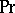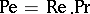# Péclet number

(diff) ← Older revision | Latest revision (diff) | Newer revision → (diff)

One of the characteristic numbers for processes of convective heat transfer. The Péclet number characterizes the relation between the convective and molecular heat-transport processes in a flow of liquid:whereis the characteristic linear scale of the heat-transfer surface,is the velocity of the liquid relative to that surface,is thermal diffusion coefficient,is the heat capacity at constant pressure,is the density, andis the thermal conductivity coefficient.

The Péclet number is related to the Reynolds numberand the Prandtl numberby.

It is named after J. Péclet.

How to Cite This Entry:
Péclet number. Encyclopedia of Mathematics. URL: http://encyclopediaofmath.org/index.php?title=P%C3%A9clet_number&oldid=12644
This article was adapted from an original article by Material from the article "PÃ©clet number" in BSE-3 (originator), which appeared in Encyclopedia of Mathematics - ISBN 1402006098. See original article